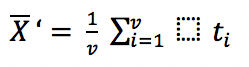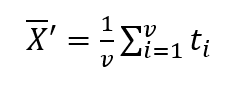# AsciiMath support

Metanorma uses the Ruby AsciiMath parser to process AsciiMath, converting it to MathML for Metanorma processing. For rendering in HTML, the MathML generated is processed by MathJax. For rendering in Word, the MathML is converted to Office Open XML (OOXML).

## Ruby AsciiMath

The version of AsciiMath supported by the Ruby AsciiMath parser is slightly different to other variants of AsciiMath. The following are differences between Ruby AsciiMath and the version of AsciiMath documented on asciimath.org:

### Miscellaneous symbols:

Ruby AsciiMath adds the following as distinct symbols:

dx

dx identifier

dy

dy identifier

dz

dz identifier

dt

dt identifier

…​

…​ operator

"\ "

non-breaking space

qquad

four non-breaking spaces (just as quad is processed as two non-breaking spaces)

lim

lim operator

Lim

Lim operator

'

prime operator, ′

:'

/_\

frown

### Relation symbols

Ruby AsciiMath adds the following as distinct symbols:

:=

:= operator

### Operation symbols

setminus

\

divide

÷

:|:

| as operator

No difference.

### Grouping brackets

Ruby AsciiMath adds the following as distinct brackets:

|:

| as left bracket

:|

| as right bracket

No difference.

### Accents

The following operators are currently missing from Ruby AsciiMath:

• color(red)(x)

• cancel(x)

Ruby AsciiMath adds the following as distinct accents

tilde

~

overarc

### Greek letters

The Ruby AsciiMath gem supports several capital letter symbols, which are not named in asciimath.org:

Alpha

Alpha

Beta

Beta

Epsilon

Epsilon

Zeta

Zeta

Eta

Eta

Iota

Iota

Kappa

Kappa

Mu

Mu

Nu

Nu

Rho

Rho

Tau

Tau

Upsilon

Upsilon

Chi

Chi

No difference.

### Font commands

The following are font commands supported by the Ruby AsciiMath gem, in addition to those named in asciimath.org:

ii

italic font

bii

bold italic font

bcc

bold script font

bfr

bold fraktur font

bsf

bold sans serif font

sfi

italic sans serif font

sfbi

sans serif bold italic font

## Conversion to Word OOXML

AsciiMath and MathJax processors are tolerant of loose syntax in mathematical expressions. Currently the conversion of MathML to OOXML is not as tolerant: if a mathematical operator takes a single argument, the OOXML expects to find that argument brackets as a single entity. If that does not happen, Word displays a dotted square where it would expect the single argument to appear. To remedy this, you will need to bracket the argument of the operator in invisible brackets ({:, :}).

For example, the following AsciiMath displays correctly in HTML under MathJax:

[stem]
++++
bar X' = (1)/(v) sum_(i = 1)^v t_i
++++

However, it displays incorrectly in Word with an extraneous “square”:That is because the sum operator expects to find a single expression as its argument, but t_i is processed as two tokens. To get this expression processed correctly for Word, you will need to bracket t_i:

[stem]
++++
bar X' = (1)/(v) sum_(i = 1)^v {:t_i:}
++++

and it should display correctly as: